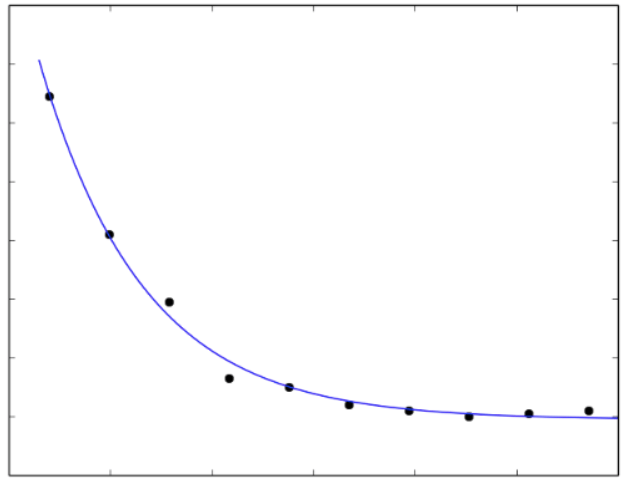# Curve-Fitting in the PSLab Android App

One of the key features of PSLab is the Oscilloscope. An oscilloscope allows observation of temporal variations in electrical signals. Its main purpose is to record the input voltage level at highly precise intervals and display the acquired data as a plot. This conveys information about the signal such as the amplitude of fluctuations, periodicity, and the level of noise in the signal. The Oscilloscope helps us to observe varying the waveform of electronic signals, it is obvious it measures a series of data points that need to be plotted on the graph of the instantaneous signal voltage as a function of time.

When periodic signals such as sine waves or square waves are read by the Oscilloscope, curve fitting functions are used to construct a curve that has the best fit to a series of data points. Curve fitting is also used on data points generated by sensors, for example, a damped sine fit is used to study the damping of the simple pendulums. The curve fitting functions are already written in Python using libraries like numpy and scipy. analyticsClass.py provides almost all the curve fitting functions used in PSLab. For the Android, implementation we need to provide the same functionality in Java.

Technically speaking, Curve-fitting is the process of constructing a curve or mathematical function, that has the best fit to a series of data points, possibly subject to constraints.

Let’s understand it with an example.Exponential Fit

The dots in the above image represent data points and the line represents the best curve fit.

In the image, data points are been plotted on the graph. An exponential fit to the given series of data can be used as an aid for data visualization. There can be many types of curve fits like sine fit, polynomial fit, exponential fit, damped sine fit, square fit etc.

### Steps to convert the Python code into Java code.

#### 1. Decoding the code

At first, we need to identify and understand the relevant code that exists in the PSLab Python project. The following is the Python code for exponential fit.

```import numpy as np
def func(self, x, a, b, c):
return a * np.exp(-x/ b) + c```

This is the model function. It takes the independent variable ie. x as the first argument and the parameters to fit as separate remaining arguments.

```def fit_exp(self, t, v):
from scipy.optimize import curve_fit
size = len(t)
v80 = v * 0.8
for k in range(size - 1):
if v[k] < v80:
rc = t[k] / .223
break
pg = [v, rc, 0]```

Here, we are calculating the initial guess for the parameters.

```po, err = curve_fit(self.func, t, v, pg)
```

curve_fit function is called here where model function func, voltage array v, time array t and a list of initial guess parameters pg are the parameters.

```if abs(err) > 0.1:
return None, None
vf = po * np.exp(-t/po) + po
return po, vf
```

#### 2. Curve-fitting in Java

The next step is to implement the functionalities in Java. The following is the code of exponential fit written in JAVA using Apache maths commons API.

```ParametricUnivariateFunction exponentialParametricUnivariateFunction = new ParametricUnivariateFunction() {
@Override
public double value(double x, double... parameters) {
double a = parameters;
double b = parameters;
double c = parameters;
return a * exp(-x / b) + c;
}
@Override
public double[] gradient(double x, double... parameters) {
double a = parameters;
double b = parameters;
double c = parameters;
return new double[]{
exp(-x / b),
(a * exp(-x / b) * x) / (b * b),
1
};
}
};
```

ParametricUnivariteFunction is an interface representing a real function which depends on an independent variable and some extra parameters. It is the model function that we used in Python.

It has two methods that are value and gradient. Value takes an independent variable and some parameters and returns the function value.

Gradient returns the double array of partial derivatives of the function with respect to each parameter (not independent parameter x).

``` public ArrayList<double[]> fitExponential(double time[], double voltage[]) {
double size = time.length;
double v80 = voltage * 0.8;
double rc = 0;
double[] vf = new double[time.length];
for (int k = 0; k < size - 1; k++) {
if (voltage[k] < v80) {
rc = time[k] / .223;
break;
}
}
double[] initialGuess = new double[]{voltage, rc, 0};
//initialize the optimizer and curve fitter.
LevenbergMarquardtOptimizer optimizer = new LevenbergMarquardtOptimizer()

```

LevenbergMarquardtOptimizer solves a least square problem using Levenberg Marquardt algorithm (LMA).

```CurveFitter fitter = new CurveFitter(optimizer);
```

LevenbergMarquardtOptimizer is used by CurveFitter for the curve fitting.

``` for (int i = 0; i < time.length; i++)

`double[] result = fitter.fit(exponentialParametricUnivariateFunction,initialGuess);`

fit method with ParametricUnivariteFunction and guess parameters as parameters return an array of fitted parameters.

```  for (int i = 0; i < time.length; i++)
vf[i] = result * exp(-time[i] / result) + result;
return new ArrayList<double[]>(Arrays.asList(result, vf));    }
```